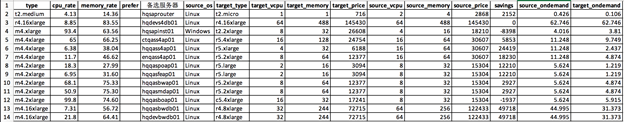# 如何自动化的选择和优化EC2系列（四）如何为SAP应用选择合适的EC2

## 方案概述

Python程序get_ec2_saps.py就可以完成这个工作：

import pandas as pd

``````import pandas as pd

``````new_table = saps_table

old_table = saps_table

new = new_table[['Instance Type', 'vCPU', 'Mem (GiB)', 'SAPS']]

old = old_table[['Instance Type', 'vCPU', 'Mem (GiB)', 'SAPS']]

ec2_saps = pd.concat([new, old])

ec2_saps.replace({'\*': ''}, regex=True, inplace=True)

ec2_saps['vCPU'] = pd.to_numeric(

ec2_saps['vCPU'], downcast='integer', errors='coerce')

ec2_saps['Mem (GiB)'] = pd.to_numeric(

ec2_saps['Mem (GiB)'], downcast='float', errors='coerce')

ec2_saps['SAPS'] = pd.to_numeric(

ec2_saps['SAPS'], downcast='float', errors='coerce')

ec2_saps.to_excel('ec2_saps.xlsx', index=False)````````````import pandas as pd

source = "blog3_output.xlsx"

sheet = 0

target = pd.ExcelWriter('result.xlsx')

for i in range(1, 6):

sheet = i

merge = ex1.merge(ex2, left_on="type",

right_on="Instance Type", how='left')

merge.drop(['Instance Type', 'vCPU', 'Mem (GiB)'], axis=1, inplace=True)

merge.rename(columns={'SAPS': 'source_saps'}, inplace=True)

merge = merge.merge(ex2, left_on="target_type",

right_on="Instance Type", how='left')

merge.drop(['Instance Type', 'vCPU', 'Mem (GiB)'], axis=1, inplace=True)

merge.rename(columns={'SAPS': 'target_saps'}, inplace=True)

merge.to_excel(target, sheet_name=str(sheet), index=False)

target.save()
``````

https://github.com/shaneliuyx/awscnprice/tree/master/examples

————

## 本篇作者### 刘育新

AWS ProServe 团队高级顾问，长期从事企业客户入云解决方案的制定和项目的实施工作。